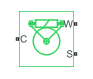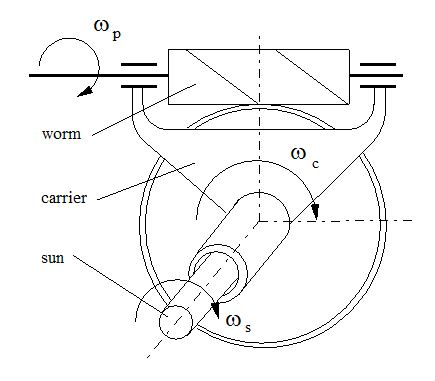# Sun-Planet Worm Gear

Planetary gear set of carrier, worm planet, and sun wheels with adjustable gear ratio, worm thread type, and friction losses

•Libraries:
Simscape / Driveline / Gears / Planetary Subcomponents

## Description

The Sun-Planet Worm Gear block represents a two-degree-of-freedom planetary gear built from a carrier and sun and planet gears. By type, the sun and planet gears are crossed helical spur gears arranged as a worm drive, in which the planet gear is the worm. Such transmissions are used in the Torsen® T-1 differential. When transmitting power, the sun gear can be independently rotated by the worm (planet) gear, by the carrier, or by both.You specify a fixed gear ratio, which signifies the worm angular velocity divided by the sun gear angular velocity. You control the direction by setting the worm thread type to left-hand or right-hand. Rotation of the right-hand worm in the positive direction causes the sun gear to rotate in the positive direction. The positive directions of the sun gear and the carrier are the same.

### Thermal Model

You can model the effects of heat flow and temperature change by enabling the optional thermal port. To enable the port, set Friction model to ```Temperature-dependent efficiency```.

### Equations

Variables

Equation variables are:

 RWG Gear ratio that signifies the worm angular velocity divided by the sun gear angular velocity: The ratio is positive for the right-hand worm and negative for the left-hand worm ωS Angular velocity of the sun gear ωP Angular velocity of the worm gear ωC Angular velocity of the carrier ωSC Angular velocity of the sun gear with respect to the carrier α Normal pressure angle λ Worm lead angle L Worm lead d Worm pitch diameter τS Torque applied to sun shaft τP Torque applied to planet shaft τC Torque applied to carrier shaft τ Torque due to meshing friction: The loss depends on the device efficiency and the power flow direction. To avoid an abrupt change of the friction torque at ωS = 0, the friction torque is introduced via the hyperbolic function. τinstfr Instantaneous value of the friction torque used to simulate friction losses τfr Torque due to friction in steady-state k Friction coefficient ηWG Efficiency of worm to gear power transfer ηGW Efficiency of gear to worm power transfer pth Power threshold μSC Viscous friction coefficient for the sun-carrier interface μWC Viscous friction coefficient for the worm-carrier interface

Ideal Gear Constraints and Gear Ratio

The Sun-Planet Worm Gear block imposes one kinematic constraint on the three connected axes:

`${\omega }_{\text{S}}=\frac{{\omega }_{\text{P}}}{{R}_{\text{WG}}}+{\omega }_{\text{C}}.$`

The gear has two independent degrees of freedom. The gear pair is (1,2) = (S,P).

The torque transfer is:

In the ideal case where there is no torque loss, τloss = 0.

Nonideal Gear Constraints

In the nonideal case, τloss ≠ 0. For more information, see Model Gears with Losses.

In a nonideal gear, the angular velocity and geometric constraints are unchanged, but the transferred torque and power are reduced by:

• Coulomb friction due to worm-sun gear meshing, which is characterized by the friction coefficient k or constant efficiencies [ηWG, ηGW]

• Viscous couplings of driveshafts with bearings, which are parametrized by viscous friction coefficients μSC and μWC

Because the transmission incorporates a worm gear, the efficiencies are different for the direct and reverse power transfer. The table shows the value of the efficiency for all combinations of the power transfer.

 Driving Shaft Driven Shaft Planet Sun Carrier Planet N/A ηWG ηWG Sun ηGW N/A No loss Carrier ηGW No loss N/A
Geometric Surface Contact Friction

When you set Friction model to ```Constant efficiency``` and leave Friction parameterization set to ```Friction coefficient and geometrical parameters```, the model considers geometric surface contact friction. In this case, ηWG and ηGW rely on:

• The worm-gear threading geometry, specified by lead angle λ and normal pressure angle α.

• The surface contact friction coefficient k.

Constant Efficiencies

When you set Friction model to ```Constant efficiency``` and set Friction parameterization to `Efficiencies`, or when you set Friction model to `Temperature-dependent efficiency`, the model treats the efficiencies as constant. In this case, you specify ηWG and ηGW independently of geometric details.

Self-Locking and Negative Efficiency

You can enable self-locking behavior by making the efficiency negative. Power cannot be transmitted from the sun gear to the worm or from the carrier to the worm unless some torque is applied to the worm to release the train. In this case, the absolute value of the efficiency specifies the ratio at which the train is released. The smaller the train lead angle, the smaller the reverse efficiency.

Meshing Efficiency

The efficiencies, η, of meshing between the worm gear and planet gear are fully active only if the transmitted power is greater than the power threshold.

If the power is less than the threshold, the actual efficiency is automatically regularized to unity at zero velocity.

Viscous Friction Force

The viscous friction coefficients of the worm-carrier and sun-carrier bearings control the viscous friction torque experienced by the carrier from lubricated, nonideal gear threads. For details, see Nonideal Gear Constraints.

### Variables

Use the Variables settings to set the priority and initial target values for the block variables before simulating. For more information, see Set Priority and Initial Target for Block Variables.

### Assumptions and Limitations

• Gear inertia is assumed to be negligible.

• Gears are treated as rigid components.

## Ports

### Conserving

expand all

Rotational mechanical conserving port associated with the planet gear carrier.

Rotational mechanical conserving port associated with the worm gear.

Rotational mechanical conserving port associated with the sun gear.

Thermal conserving port associated with heat flow. Heat flow affects the power transmission efficiency by altering the gear temperatures.

#### Dependencies

To enable this port, set Friction model to `Temperature-dependent efficiency`.

## Parameters

expand all

### Main

Gear or transmission ratio, RWG, which signifies the worm gear angular velocity divided by the sun gear angular velocity. This gear ratio must be strictly positive.

Direction of positive worm rotation. If you select `Left-hand`, positive worm rotation results in negative gear rotation.

### Meshing Losses

The table shows how the options that you choose for Friction model affect the visibility of other parameters in the Meshing Losses tab. To learn how to read the table, see Parameter Dependencies.

Meshing Losses Parameter Dependencies

Meshing Losses Setting Parameters and Values
Friction Model
```No meshing losses - Suitable for HIL simulation``````Constant efficiency``````Temperature-dependent efficiency```
Friction parameterizationTemperature
```Friction coefficient and geometrical parameters````Efficiencies`
Normal pressure angleWorm-gear efficiencyWorm-gear efficiency
Friction coefficient
Power thresholdPower thresholdPower threshold

Friction model for the block:

• `No meshing losses - Suitable for HIL simulation` — Gear meshing is ideal.

• `Constant efficiency` — Transfer of torque between the gear wheel pairs is reduced by a constant efficiency, η, such that 0 < η ≤ 1.

• `Temperature-dependent efficiency` — Transfer of torque between the gear wheel pairs is defined by the table lookup based on the temperature.

Characterization of the friction between gear threads:

• ```Friction coefficient and geometrical parameters``` — Friction is determined by geometric surface contact friction.

• `Efficiencies` — Friction is determined by constant efficiencies, where 0 < η < 1.

#### Dependencies

To enable this parameter, set Friction model to `Constant efficiency`.

Thread pressure angle, α, in the normal plane. The value must be greater than zero and less than 90 degrees.

#### Dependencies

To enable this parameter, set Friction model to `Constant efficiency` and Friction parameterization to ```Friction coefficient and geometrical parameters```.

Thread helix angle, where λ = arctan[L/(πd)]. L is the worm lead and d is the worm pitch diameter. This value must be greater than zero.

#### Dependencies

To enable this parameter, set Friction model to `Constant efficiency` and Friction parameterization to ```Friction coefficient and geometrical parameters```.

Dimensionless coefficient of normal friction in the thread. Must be greater than zero.

#### Dependencies

To enable this parameter, set Friction model to `Constant efficiency` and Friction parameterization to ```Friction coefficient and geometrical parameters```.

Vector of temperatures used to construct a 1-D temperature-efficiency lookup table. The vector elements must increase from left to right.

#### Dependencies

To enable this parameter, set Friction model to `Temperature-dependent efficiency`.

Vector of output-to-input power ratios that describe the power flow from the worm gear to the sun gear, ηWG. When you set Friction model to ```Constant efficiency```, specify the value as a scalar. When you set Friction model to `Temperature-dependent efficiency`, specify the value as a vector. The block uses the vector values to construct a 1-D temperature-efficiency lookup table.

Each element is an efficiency that relates to a temperature in the Temperature vector. The length of the vector must be equal to the length of the Temperature vector. Each element in the vector must be in the range (0,1].

#### Dependencies

To enable this parameter, set either:

• Friction model to `Constant efficiency` and Friction parameterization to `Efficiencies` — In this case, specify the value as a scalar.

• Friction model to ```Temperature-dependent efficiency``` — In this case, specify the value as a vector.

Vector of output-to-input power ratios that describe the power flow from the sun gear to the worm gear, ηGW. When you set Friction model to ```Constant efficiency```, specify the value as a scalar. When you set Friction model to `Temperature-dependent efficiency`, specify the value as a vector. The block uses the vector values to construct a 1-D temperature-efficiency lookup table.

Each element is an efficiency that relates to a temperature in the Temperature vector. The length of the vector must be equal to the length of the Temperature vector. Each element in the vector must be in the range (0,1].

#### Dependencies

To enable this parameter, set either:

• Friction model to `Constant efficiency` and Friction parameterization to `Efficiencies` — In this case, specify the value as a scalar.

• Friction model to ```Temperature-dependent efficiency``` — In this case, specify the value as a vector.

Power threshold, pth, above which full efficiency is in effect. Below this value, a hyperbolic tangent function smooths the efficiency factor.

When you set Friction model to `Constant efficiency`, the block lowers the efficiency losses to zero when no power is transmitted. When you set Friction model to `Temperature-dependent efficiency`, the function smooths the efficiency factors between zero when at rest and the values provided by the temperature-efficiency lookup tables at the power thresholds.

#### Dependencies

To enable this parameter, set Friction model to `Constant efficiency` or ```Temperature-dependent efficiency```.

### Viscous Losses

Vector of viscous friction coefficients [μWC μSC], for the worm-carrier and sun-carrier shafts, respectively.

### Thermal Port

To enable this parameter, set Friction model to `Temperature-dependent efficiency`.

Thermal energy required to change the component temperature by a single temperature unit. The greater the thermal mass, the more resistant the component is to temperature change.

#### Dependencies

To enable this parameter, set Friction model to ```Temperature-dependent efficiency```.

expand all

## Version History

Introduced in R2011a